# SRCNN论文翻译（Image Super-Resolution Using Deep Convolutional Networks）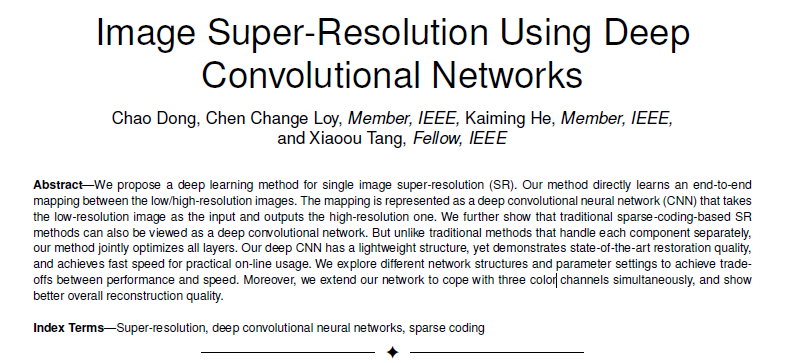## 1 INTRODUCTION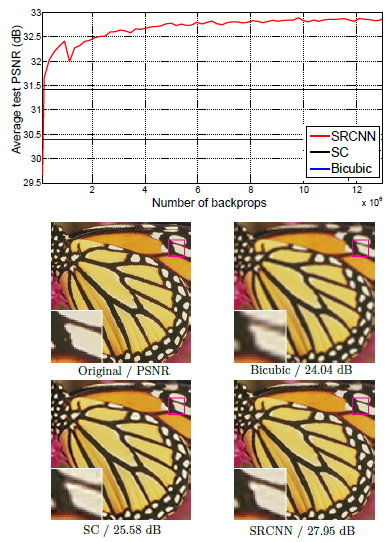## 3 CONVOLUTIONAL NEURAL NETWORKS FOR SUPER-RESOLUTION

### 3.1 Formulation

1）补丁提取和表示：该操作从低分辨率图像Y中提取（重叠）补丁，并将每个补丁表示为高维向量。这些向量包括一组特征图，其数量等于向量的维数。
2）非线性映射：该操作是每个高维向量到另一个高维向量的非线性映射。每个映射的矢量在概念上是高分辨率补丁的表示。这些向量包括另一组特征映射。
3）重建：该操作聚合高分辨率补丁表示以生成最终的高分辨率图像。预计该图像与地面实况X相似。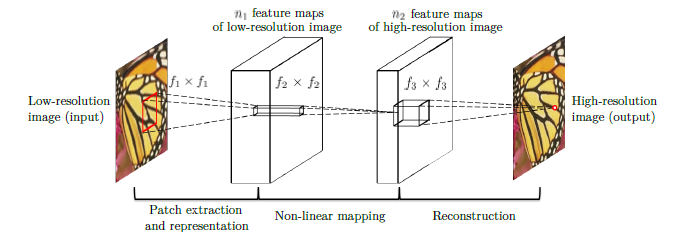### 3.1.1 Patch extraction and representation

F 1 ( Y ) = max ⁡ ( 0 , W 1 ∗ Y + B 1 ) F_{1}(\mathbf{Y})=\max \left(0, W_{1} * \mathbf{Y}+B_{1}\right)

### 3.1.2 Non-linear mapping 非线性映射

F 2 ( Y ) = max ⁡ ( 0 , W 2 ∗ F 1 ( Y ) + B 2 ) F_{2}(\mathbf{Y})=\max \left(0, W_{2} * F_{1}(\mathbf{Y})+B_{2}\right)

### 3.1.3 Reconstruction

F ( Y ) = W 3 ∗ F 2 ( Y ) + B 3 F(\mathbf{Y})=W_{3} * F_{2}(\mathbf{Y})+B_{3}

### 3.2 Relationship to Sparse-Coding-Based Methods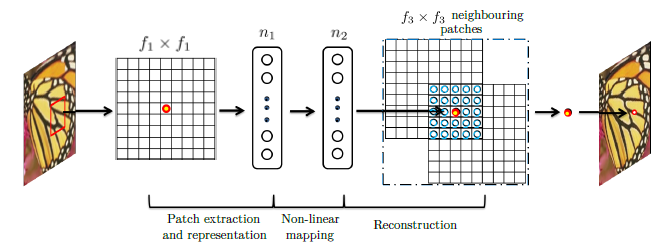### 3.3 Training

L ( Θ ) = 1 n ∑ i = 1 n ∥ F ( Y i ; Θ ) − X i ∥ 2 L(\Theta)=\frac{1}{n} \sum_{i=1}^{n}\left\|F\left(\mathbf{Y}_{i} ; \Theta\right)-\mathbf{X}_{i}\right\|^{2}

Δ i + 1 = 0.9 ⋅ Δ i + η ⋅ ∂ L ∂ W i ℓ , W i + 1 ℓ = W i ℓ + Δ i + 1 \Delta_{i+1}=0.9 \cdot \Delta_{i}+\eta \cdot \frac{\partial L}{\partial W_{i}^{\ell}}, \quad W_{i+1}^{\ell}=W_{i}^{\ell}+\Delta_{i+1}

## 4 EXPERIMENTS

### 4.1 Training Data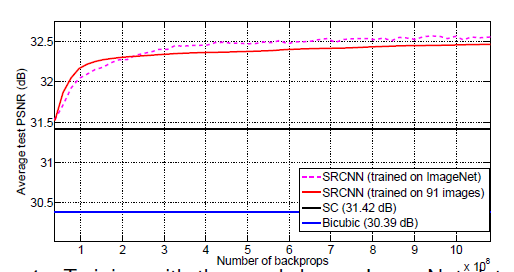### 4.2 Learned Filters for Super-Resolution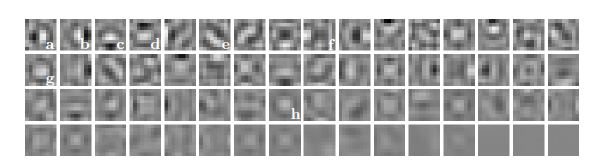### 3.与最前沿的其他超分算法对比速度与性能的实验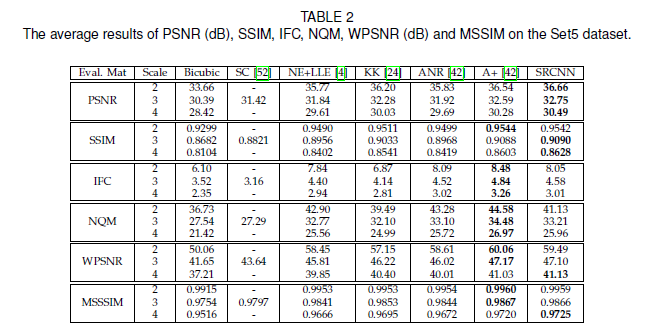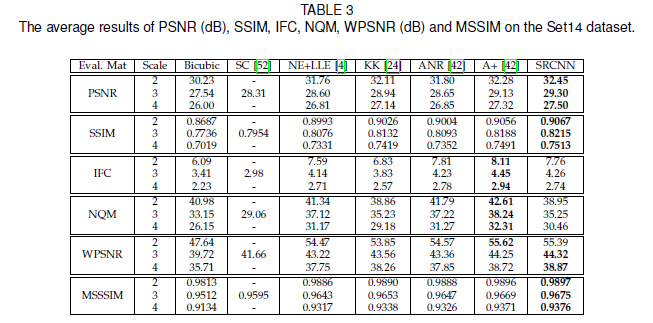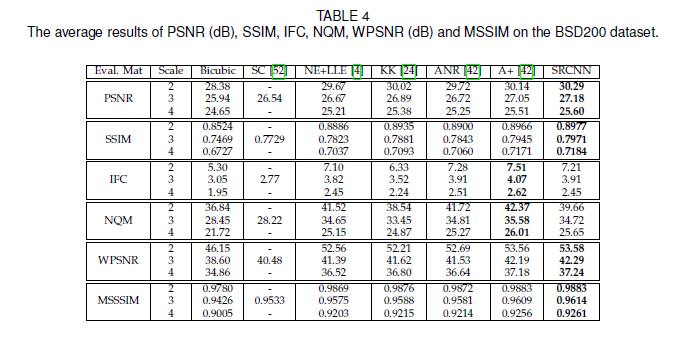## CONCLUSION11-24
06-112581
05-181850
05-308310
01-21579
07-25
11-24
01-242081
03-12
12-08386
05-312万+
01-041281
04-0484
05-234641
11-124011
07-01
02-219798
10-28
©️2020 CSDN 皮肤主题: 创作都市 设计师:CSDN官方博客